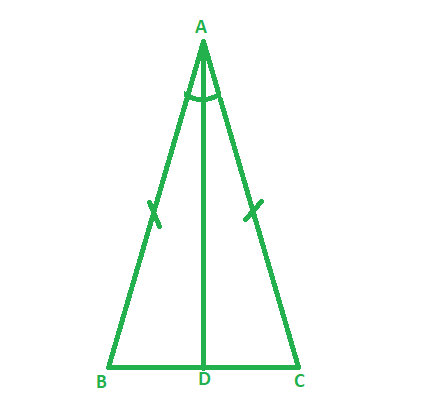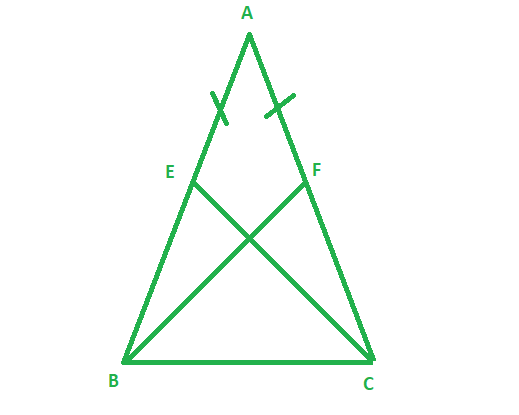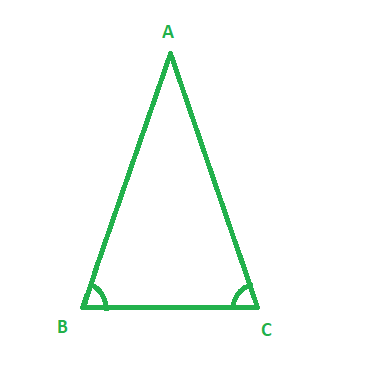Open in App
Not now

# Theorem – Angle opposite to equal sides of an isosceles triangle are equal | Class 9 Maths

• Difficulty Level : Hard
• Last Updated : 15 Dec, 2020

In geometry, an isosceles triangle is a triangle that has two sides of equal length. Sometimes it is specified as having exactly two sides of equal length, and sometimes as having at least two sides of equal length, the latter version thus including the equilateral triangle as a special case. Examples of isosceles triangles include the isosceles right triangle, the golden triangle, and the faces of bipyramids and certain Catalan solids.

### Theorem Statement: Angle opposite to equal sides of an isosceles triangle are equal.

Proof:Given, an Isosceles triangle ABC, where the length of side AB equals the length of side AC.

Therefore, AB = AC

Construction:

Let us draw the bisector of ∠A

Let D be the point of intersection of this bisector of ∠A and BC.

AB = AC (Given)

So, ∠ABD = ∠ACD, since they are corresponding angles of congruent triangles.

So, ∠B = ∠C

Hence, Proved that an angle opposite to equal sides of an isosceles triangle is equal.

Note:

The converse of this theorem is also true. The sides opposite to equal angles of a triangle are also equal.

## Sample Problems Based on the Theorem

### Problem 1: E and F are respectively the mid-points of equal sides AB and AC of ∆ABC (see given figure). Show that BF = CE.Solution:

Given:

Length of side AB = AC

To show: BF = CE

In  ∆ABF and ∆ACE,

AB = AC (Given)

∠A =  ∠A (Common)

AF = AE (Halves of equal sides)

So, ∆ABF ≅ ∆ACE (SAS rule)

Since, If two triangles are congruent, their corresponding sides are equal.

Therefore, BF = CE ( by CPCT)

### Problem 2: Given an ∆ABC whose perimeter is 13 cm and ∠ABC = ∠ACB and length of side BC equals 3 cm. Find the length of side AB and AC.Solution:

Given:

BC = 3cm, Perimeter of   ∆ABC = 13cm

∠ABC = ∠ACB

Since ∠ABC = ∠ACB , therefore by applying theorem, the sides opposite to equal angles of a triangle are also equal.

So, length of side AB = AC.

Let the side of AB be x.

Therefore, Perimeter = AB + BC + AC

13 = x + 3 + x ( Since, AB = AC )

13 = 2x + 3

13 – 3 = 2x

10/2 = x

Therefore x = 5

So, the length of side AB and AC is 5 cm.

My Personal Notes arrow_drop_up
Related Articles